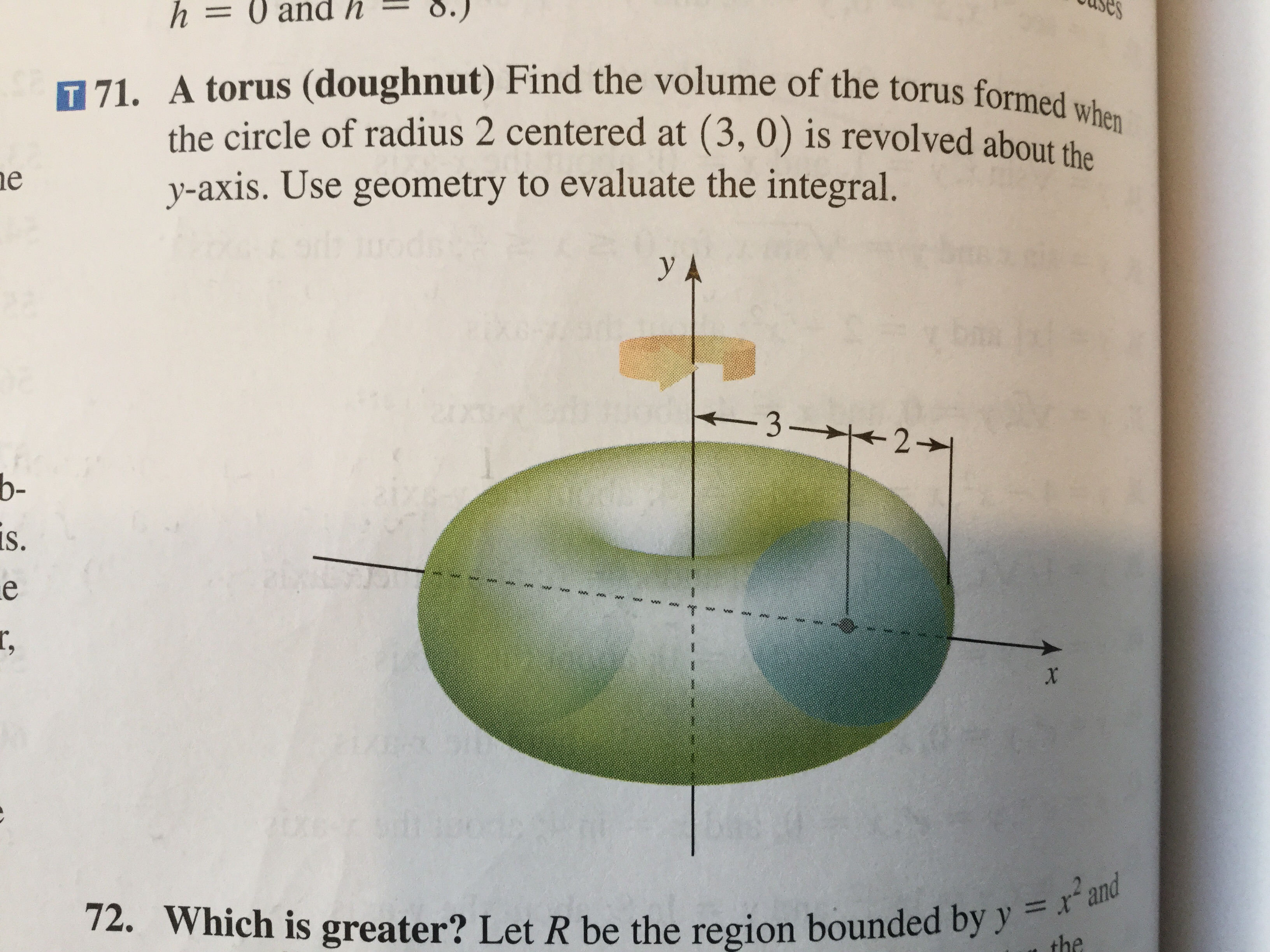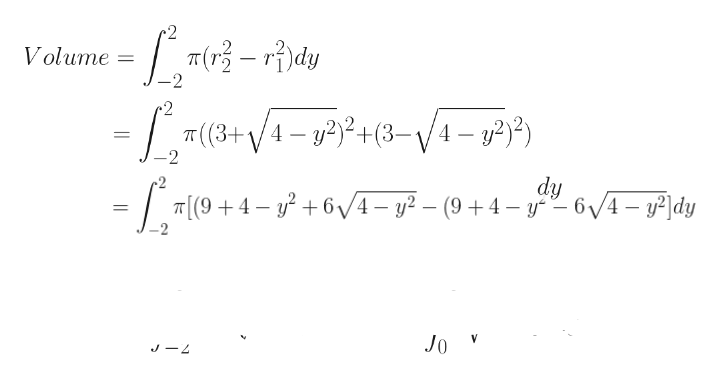# esh= 0 and hT 71. A torus (doughnut) Find the volume of the torus formed whenthe circle of radius 2 centered at (3, 0) is revolved about thay-axis. Use geometry to evaluate the integral.ey A-3 21b-is.er,x2U6072. Which is greater? Let R be the region bounded by y = r'and=the

Question
14 views

I need help on 71.help_outlineImage Transcriptionclosees h= 0 and h T 71. A torus (doughnut) Find the volume of the torus formed when the circle of radius 2 centered at (3, 0) is revolved about tha y-axis. Use geometry to evaluate the integral. e y A -3 21 b- is. e r, x 2U60 72. Which is greater? Let R be the region bounded by y = r'and = the fullscreen
check_circle

Step 1

Equation fo the circle centred at (3, 0) and with radius 2 will be:

(x – 3)2 + (y – 0)2 = 22 = 4

Or, (x – 3)2 + y2 = 4

Step 2help_outlineImage TranscriptioncloseT(-rdy Volume 2 /4- P) # (3+ /4- 22+(3-\/4-) - 2 2 dy 6/4- y2 - (9 4- y6/4-y2dy T[(94 Jo J4 fullscreen
Step 3

Now the geometry can be used to evaulate this integral as per any of the two methods below:

Method 1: The area under integral is nothing but the area of the one quarte...

### Want to see the full answer?

See Solution

#### Want to see this answer and more?

Solutions are written by subject experts who are available 24/7. Questions are typically answered within 1 hour.*

See Solution
*Response times may vary by subject and question.
Tagged in

### Integration